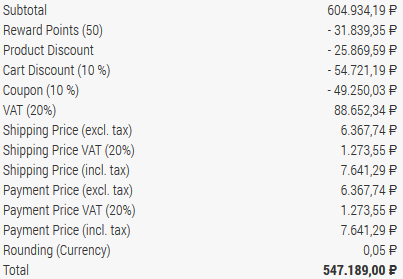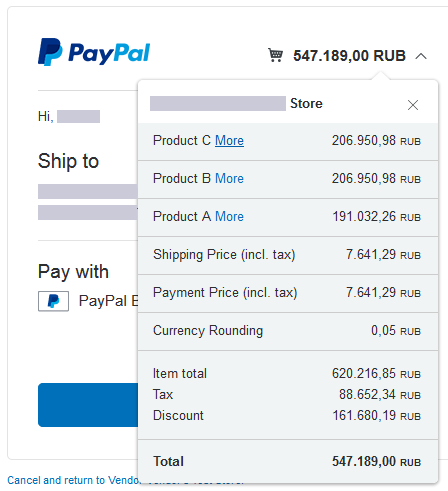## Phoca Cart - Development

Category: Phoca Cart Component

### Phoca Cart Payment Plugin

There are peculiarities you need to care about when developing a payment plugin. Calculation used in the cart can differ from calculation used in payment method. Mostly this is because of rounding and usage of foreign currency. To understand the following examples, we need to explain some terms used in this guide:

• Cart Calculation - calculation of items in our e-shop
• Payment Method Calculation - calculation of items in payment method (payment gateway)
• Default Currency - default currency set as default in our e-shop (currency rate is 1)
• Order Currency - foreign currency (other than default) which was selected and used by customer when making an order (currency rate is not 1)

#### Examples of problematic parts in the payment methods

Example 1: PayPal Standard payment method calculation differs from cart calculation:

• The total amount in cart calculation is calculated as follows: (product * quantity) * currency rate
• The total amount in payment calculation is calculated as follows: (product * currency rate) * quantity

There are many reasons why cart calculation uses a different calculation model than payment calculation. This happens because of calculation of reward points discount, product discount, cart discount, coupon. Because of storing all items in the same currency. Because of recalculating taxes (fixed amount or percentage), etc.

Example 2: Rounding:

• Some payment methods do not support rounding so it needs to be added as an extra item. Rounding value is different for each currency. When making a complete calculation, rounding calculation may vary. This is why the total amount needs to be compared (cart total amount vs. payment method total amount) and the difference must be set as rounding.

Example 3: Missing items

• Some payment methods do not support certain items: Rounding, different discounts, etc. This is why we need to add different items to one variable (one item displayed in payment method calculation). For example, in Paypal Standard payment method, there is only one item for discount. So we need to add all discounts and all negative values into one variable.

#### Code example

To see full code, just open the default PayPal Standard plugin which is included in Phoca Cart ZIP installation package. The example code parts listed here are simplified, mostly there are more rows for one item.

1. Define variables

``````\$cartBrutto      = 0;// Total amount (brutto) calculated by cart
\$paymentBrutto   = 0;// Total amount (brutto) calculated by payment method
\$discountAmount  = 0;// Sum of all discount values - all MINUS values
\$currencyAmount  = 0;// Sum of all currency rounding amounts - all PLUS values``````

At the end of the calculation we need to compare cart total amount and payment method total amount. Both must be equal - in the payment gateway customer should pay the same price which was displayed in calculation of our e-shop. If the values are different, we need to set this difference as rounding. Rounding can have positive value (PLUS) or negative value (MINUS). In our example, PayPal Standard doesn't have any specific item for rounding. So when rounding is positive (PLUS) we need to add it to payment method calculation as a new plus item. When rounding is negative (MINUS) we need to add it to discount item. In PayPal Standard, there is only one discount item, so we need to join all discounts to one item.

2. Sum of all product prices (PLUS)

``````foreach (\$order['products'] as \$k => \$v) {
\$paymentBrutto = \$paymentBrutto + ((\$price->roundPrice(\$v->netto * \$r)) * (int)\$v->quantity);
echo '';
}``````

First, we need to add product price of each product to \$paymentBrutto variable (payment method total amount). We compare this amount with cart total amount at the end. Second we need to add the items to payment system. For example, PayPal Standard payment method uses a form which will be sent to PayPal payment gateway. So we add each product price into the form input field.

3. Sum of all total values (PLUS/MINUS)

``````foreach (\$order['total'] as \$k => \$v) {
if (\$v->amount != 0 || \$v->amount_currency != 0) {

switch(\$v->type) {

// All discounts (MINUS)
case 'dnetto':
\$paymentBrutto       += \$price->roundPrice(\$v->amount * \$r);
\$discountAmount 	+= \$price->roundPrice(abs(\$v->amount * \$r));
break;

// Tax (PLUS)
case 'tax':
\$paymentBrutto       += \$price->roundPrice(\$v->amount * \$r);
\$f[]	= '';
break;

// Payment Method, Shipping Method (PLUS)
case 'sbrutto':
case 'pbrutto':
\$paymentBrutto       += \$price->roundPrice(\$v->amount * \$r);
\$f[]	= '';

break;

// Rounding (PLUS/MINUS)
case 'rounding':
if (\$v->amount_currency != 0) {
// Rounding is set in order currency
if (\$v->amount_currency > 0) {
\$currencyAmount      += round(\$v->amount_currency, 2, \$rounding_calculation);
\$paymentBrutto       += round(\$v->amount_currency, 2, \$rounding_calculation);
} else if (\$v->amount_currency < 0) {
\$discountAmount 	+= round(abs(\$v->amount_currency), 2, \$rounding_calculation);
\$paymentBrutto       += round(\$v->amount_currency, 2, \$rounding_calculation);
}
} else {
// Rounding is set in default currency
if (\$v->amount > 0 && round((\$v->amount * \$r), 2, \$rounding_calculation) > 0) {

\$f[]	= '';
\$paymentBrutto       += round((\$v->amount * \$r), 2, \$rounding_calculation);
} else if (\$v->amount < 0) {
\$discountAmount 	+= round(abs(\$v->amount * \$r), 2, \$rounding_calculation);
\$paymentBrutto       += round((\$v->amount * \$r), 2, \$rounding_calculation);
}
}
break;

// Brutto (total amount)
case 'brutto':
if (\$v->amount_currency != 0) {
// Brutto is set in order currency
\$cartBrutto = \$price->roundPrice(\$v->amount_currency);
} else {
// Brutto is set in default currency
\$cartBrutto = \$price->roundPrice(\$v->amount * \$r);
}
break;
}
}
}``````
Type Description
dnetto All discounts: reward points discount, product discount, cart discount, coupon. Discounts have a negative value (MINUS). When adding them to \$paymentBrutto variable, the value will be deducted. Because PayPal Standard payment method has only one item for discount, we need to add all the discount values to one variable \$discountAmount. This variable will be added to the form at the end as one item
tax Tax is added to variable \$paymentBrutto. And it is added to the payment form.
sbrutto, pbrutto Shipping and payment costs are added to variable \$paymentBrutto. And they are added to payment form.
rounding First of all we need to differentiate between currencies. If the calculation is done in order currency then we use \$v->amount_currency, if it is done in default currency, then we use \$v->amount. Next we need to detect if the rounding has positive (PLUS) or negative value (MINUS). Because there is no specific item in PayPal Standard payment method for adding rounding, we need to add it to the calculation as new item (\$currencyAmount) in case the value is positive (PLUS) or we need to add it to the variable \$discountAmount in case the value is negative (MINUS).
brutto We only store this information into variable \$cartBrutto so we can compare our cart brutto value (cart total amount) with the payment brutto value (payment total amount). The difference will be set as rounding value.

Be aware whether the amount is set in default currency or order currency (foreign currency). The amount set in default currency value is multiplied by currency rate of the order currency. The amount set in order currency (foreign currency) is no more multiplied by currency rate.

4. Compare cart total amount and payment total amount

``````if (\$cartBrutto > \$paymentBrutto) {

// in PayPal - if currency rounding plus then make new item
\$currencyAmount		+= (\$cartBrutto - \$paymentBrutto);

} else if (\$cartBrutto < \$paymentBrutto) {

// in PayPal - if currency rounding minus then make it as a part of discount
\$discountAmount 	+= (\$paymentBrutto - \$cartBrutto);
}``````

Here we compare the total amount of cart and the total amount of payment method. If the rounding is positive (PLUS) then it is added to \$currencyAmount variable. If it is negative (MINUS) then it is added to \$discountAmount variable.

5. Add the discount amount and currency amount to the form

``````if (round(\$discountAmount, 2, \$rounding_calculation) > 0) {
echo '';
}

if (round(\$currencyAmount, 2, \$rounding_calculation) > 0) {
echo '';
}``````

At the end we add the variables \$discountAmount and \$currencyAmount to the payment form.

Summarization

We count all items and all the total values. Because cart calculation differs from payment calculation, we compare payment calculation sum with sum made in our cart. If it differs then we create new item for rounding. If rounding value is positive (PLUS) we create a new plus item for the payment method, if it is negative (MINUS) we add this value to the discount variable (discount variable is sum of all discounts and negative values).

See images:

1. Cart calculation set in our e-shop:Cart calculation set in our e-shop

1. Cart calculation set in payment method:Cart calculation set in payment method

As you can see, PayPal Standard payment method has only one item for discount values. So we add all discounts including all negative values (e.g. rounding) into one item called Discount. In our case the rounding value is positive (PLUS), so we created a new plus item for rounding.

Each payment method can have different settings. So e.g. in some payment methods, you don't need to collect all discounts and negative values into one item and you can add them to the form separately. Some don't have individual items, so you can add total amounts only without checking the cart total amount and payment method total amount. If you plan to develop new payment method, the best way is to see the PayPal Standard plugin inside Phoca Cart ZIP installation package. To get more information, just ask in Phoca Forum.

### Events and Plugins in Phoca Cart

There are 4 groups of plugins in Phoca Cart:

• pcp - payment
• pcs - shipping
• pcv - view
• pcf - feed

Events are assigned to these plugins but there are also general events used e.g. in system plugins.

PCP Events:

• PCPbeforeSaveOrder
• PCPbeforeProceedToPayment
• PCPafterCancelPayment
• PCPbeforeSetPaymentForm
• PCPbeforeCheckPayment
• PCPonDisplayPaymentPos
• PCPonPaymentWebhook

View Events:

• PCVonCheckoutAfterCart
• PCVonCheckoutAfterShipping
• PCVonCheckoutAfterPayment
• PCVonCheckoutAfterConfirm
• PCVonItemBeforeEndPricePanel
• PCVonItemInsideTabPanel
• PCVonItemAfterTabs
• PCVonItemImage